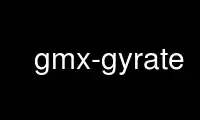# gmx-gyrate - Online in the CloudThis is the command gmx-gyrate that can be run in the OnWorks free hosting provider using one of our multiple free online workstations such as Ubuntu Online, Fedora Online, Windows online emulator or MAC OS online emulator

### PROGRAM:

#### NAME

gmx-gyrate - Calculate the radius of gyration

#### SYNOPSIS

gmx gyrate [-f [<.xtc/.trr/...>]] [-s [<.tpr/.gro/...>]] [-n [<.ndx>]]
[-o [<.xvg>]] [-acf [<.xvg>]] [-b <time>] [-e <time>]
[-dt <time>] [-[no]w] [-xvg <enum>] [-nmol <int>] [-[no]q]
[-[no]p] [-[no]moi] [-nz <int>] [-acflen <int>]
[-[no]normalize] [-P <enum>] [-fitfn <enum>]
[-beginfit <real>] [-endfit <real>]

#### DESCRIPTION

the x-, y- and z-axes, as a function of time. The atoms are explicitly mass weighted.

The axis components corresponds to the mass-weighted root-mean-square of the radii
components orthogonal to each axis, for example:

Rg(x) = sqrt((sum_i m_i (R_i(y)^2 + R_i(z)^2))/(sum_i m_i)).

With the -nmol option the radius of gyration will be calculated for multiple molecules by
splitting the analysis group in equally sized parts.

With the option -nz 2D radii of gyration in the x-y plane of slices along the z-axis are
calculated.

#### OPTIONS

Options to specify input files:

-f [<.xtc/.trr/...>] (traj.xtc)
Trajectory: xtc trr cpt gro g96 pdb tng

-s [<.tpr/.gro/...>] (topol.tpr)
Structure+mass(db): tpr gro g96 pdb brk ent

-n [<.ndx>] (index.ndx) (Optional)
Index file

Options to specify output files:

-o [<.xvg>] (gyrate.xvg)
xvgr/xmgr file

-acf [<.xvg>] (moi-acf.xvg) (Optional)
xvgr/xmgr file

Other options:

-b <time> (0)
First frame (ps) to read from trajectory

-e <time> (0)
Last frame (ps) to read from trajectory

-dt <time> (0)
Only use frame when t MOD dt = first time (ps)

-[no]w (no)
View output .xvg, .xpm, .eps and .pdb files

-xvg <enum>
xvg plot formatting: xmgrace, xmgr, none

-nmol <int> (1)
The number of molecules to analyze

-[no]q (no)
Use absolute value of the charge of an atom as weighting factor instead of mass

-[no]p (no)

-[no]moi (no)
Calculate the moments of inertia (defined by the principal axes).

-nz <int> (0)
Calculate the 2D radii of gyration of this number of slices along the z-axis

-acflen <int> (-1)
Length of the ACF, default is half the number of frames

-[no]normalize (yes)
Normalize ACF

-P <enum> (0)
Order of Legendre polynomial for ACF (0 indicates none): 0, 1, 2, 3

-fitfn <enum> (none)
Fit function: none, exp, aexp, exp_exp, exp5, exp7, exp9

-beginfit <real> (0)
Time where to begin the exponential fit of the correlation function

-endfit <real> (-1)
Time where to end the exponential fit of the correlation function, -1 is until the
end

Use gmx-gyrate online using onworks.net services